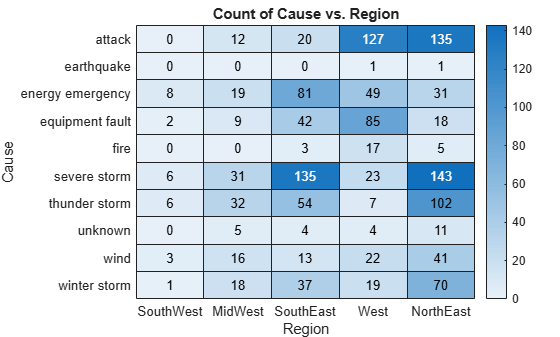# sortx

Sort elements in heatmap row

## Syntax

``sortx(h,row)``
``sortx(h,row,direction)``
``sortx(___,'MissingPlacement',lcn)``
``sortx(h)``
``C = sortx(___)``
``[C,x] = sortx(___)``

## Description

example

````sortx(h,row)` displays the elements in `row` in ascending order (from left to right). This function sorts the row elements by rearranging the columns. Specify `row` as one element or as an array of elements from `YData`. If the first row that you specify has repeating values, then `sortx` rearranges the remaining columns according to the second row, and so on. ```

example

````sortx(h,row,direction)`, where `direction` is `'descend'`, sorts the values in descending order instead of ascending order (default). To sort in a unique direction for each row in `row`, specify `direction` as an array whose elements are `'ascend'` or `'descend'`.```
````sortx(___,'MissingPlacement',lcn)` specifies whether to put `NaN` elements first or last in the sort order. Specify `lcn` as `'first'`, `'last'`, or `'auto'`. The default setting is `'auto'`, which lists `NaN` elements last for ascending order and first for descending order. Use this option after any of the previous input argument combinations.```
````sortx(h)` displays the elements in the top row in ascending order. If the first row has repeating elements, then `sortx` rearranges the remaining columns according to the second row, and so on. ```

example

````C = sortx(___)` returns the sorted color data, which is a matrix of values as they appear in the heatmap chart.```
````[C,x] = sortx(___)` also returns the sorted x values in the order they are displayed.```

## Examples

collapse all

Create a heatmap of utility outages and sort the values in a particular row so that they appear in ascending order, from left to right.

First, create a heatmap. Read the sample file `outages.csv` into a table. The sample file contains data representing electric utility outages in the United States. The table contains six columns: `Region`, `OutageTime`, `Loss`, `Customers`, `RestorationTime`, and `Cause`. Create a heatmap that shows the different regions along the x-axis and the different outage causes along the y-axis. In each cell, show how many times each region experienced a power outage due to a particular cause.

```T = readtable('outages.csv'); h = heatmap(T,'Region','Cause');```Sort the values in the `'winter storm'` row so that they appear in ascending order from left to right.

`sortx(h,'winter storm')`Create a heatmap of utility outages and display the values in the `'winter storm'` row in descending order by rearranging the columns.

```T = readtable('outages.csv'); h = heatmap(T,'Region','Cause'); sortx(h,'winter storm','descend')```Create a heatmap of utility outages and rearrange the columns using values from multiple rows. Sort the columns so that the row `'unknown'` appears in ascending order. Since that row contains duplicate values, use the row `'earthquake'` to sort the remaining columns.

```T = readtable('outages.csv'); h = heatmap(T,'Region','Cause'); sortx(h,{'unknown','earthquake'})```Create a heatmap of utility outages and sort the values in the `'winter storm'` row so that they appear in ascending order. Return the sorted color data and the column values (x values) in the order that they appear along the x-axis.

```T = readtable('outages.csv'); h = heatmap(T,'Region','Cause'); [C,x] = sortx(h,'winter storm')``````C = 10×5 0 12 127 20 135 0 0 1 0 1 8 19 49 81 31 2 9 85 42 18 0 0 17 3 5 6 31 23 135 143 6 32 7 54 102 0 5 4 4 11 3 16 22 13 41 1 18 19 37 70 ```
```x = 5x1 cell {'SouthWest'} {'MidWest' } {'West' } {'SouthEast'} {'NorthEast'} ```

Create a heatmap of utility outages and sort the values in the top row in ascending order by rearranging the columns. Then, restore the original order.

```T = readtable('outages.csv'); h = heatmap(T,'Region','Cause'); sortx(h)```Restore the original column order by setting the `XDisplayData` property of the `HeatmapChart` object equal to the `XData` property.

`h.XDisplayData = h.XData;`## Input Arguments

collapse all

Heatmap to update, specified as a `HeatmapChart` object.

Row to sort, specified as a character vector, cell array of character vectors, or string array indicating one or more elements from the `YData` or `YDisplayData` property of the `HeatmapChart` object.

Example: `sortx(h,'MyRowName')` sorts the elements in the row called `'MyRowName'` in ascending order.

Direction to sort, specified as one of these values:

• `'ascend'` — Sort all rows in `row` in ascending order.

• `'descend'` — Sort all rows in `row` in descending order.

• Array — Sort in a unique direction for each row in `row`. Specify `direction` as a cell array of character vectors or a string array containing the elements `'ascend'` or `'descend'`. The array must be the same length as `row`.

Example: `sortx(h,row,{'ascend','descend','ascend'})` sorts the elements in the first row in `row` in ascending order. If there are any repeating values in the first row, then it breaks ties using the elements of the second row in descending order, and so on.

`NaN` placement, specified as one of these values:

• `'auto'` — List `NaN` elements last for ascending order and first for descending order.

• `'first'` — List `NaN` elements first.

• `'last'` — List `NaN` elements last.

Example: `sortx(h,row,'MissingPlacement','last')`

## Output Arguments

collapse all

Sorted color data, returned as a matrix. The values in the matrix appear in the same order as they appear on the heatmap.

The `ColorDisplayData` property of the `HeatmapChart` object stores the sorted color data. The `ColorData` property stores the unsorted color data.

Rearranged column order, returned as a cell array of character vectors.

The `XDisplayData` property of the `HeatmapChart` object stores the sorted column order. The `XData` property stores the unsorted column order.

## Algorithms

The `sortx` function sets and queries these `HeatmapChart` object properties:

• `XDisplayData` — Property that stores the x-axis data.

• `ColorDisplayData` — Property that stores the color display data.

The `sortx` function also resets the `XLimits` property.

## Version History

Introduced in R2017b